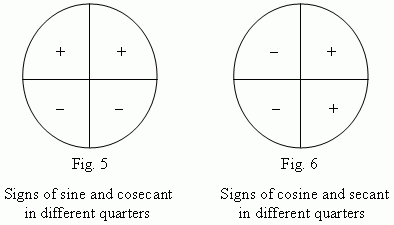If cos >0 and sin <0 what quadrant is it in?

May 29, 2016

Consider the rule $C - A - S - T$ or All Slow Turtles Crawl for this type of problem.

Both of these rules indicate in which quadrant the trigonometric function is positive. In all quadrants except the first, only 1 out of the three trigonometric functions are positive; the other two are negative.

Quadrant 1: All are positive
Quadrant 2: Sine is positive
Quadrant 3: Tangent is positive
Quadrant 4: Cosine is positive

The acronym and the expression mentioned above are meant to facilitate your ability to remember these. Beware, though that C-A-S-T starts in the 4th quadrant and then goes to the 1st, 2nd and finally the 3rd, while "All Slow Turtles Crawl# goes from 1st to 4th.

Now, back to the problem at hand.

If cosine is positive, then we are already limited to 2 quadrants: IV and I. However, in quadrant I, all the functions are positive, and the problem says that sin is negative in this case. This leaves us one option: quadrant IV.

Hopefully this helps!

May 29, 2016

$\text{Quadrant IV}$

Explanation:

Cosine correlates with values of $x$.
Values of $x$ are positive in $\text{Quadrant I}$ and $\text{Quadrant IV}$.
Cosine is positive in the same quadrants.

Sine correlates with values of $y$.
Values of $y$ are negative in $\text{Quadrant III}$ and $\text{Quadrant IV}$.
Sine is negative in the same quadrants.

The only quadrant where $x$ is positive, so $\cos \left(x\right) > 0$, and $y$ is negative, so $\sin \left(x\right) < 0$, is $\text{Quadrant IV}$.An example of an angle in $\text{Quadrant 4}$ is $\frac{7 \pi}{4}$.

$\cos \left(\frac{7 \pi}{4}\right) = \frac{\sqrt{2}}{2} > 0$

$\sin \left(\frac{7 \pi}{4}\right) = - \frac{\sqrt{2}}{2} < 0$